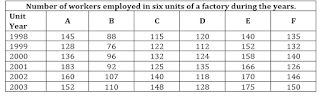# Quantitative Aptitude for NIACL Assistant Prelims Exam: 31st August 2018

Dear students,

Numerical Ability or Quantitative Aptitude Section has given heebie-jeebies to the aspirants when they appear for a banking examination. As the level of every other section is only getting complex and convoluted, there is no doubt that this section, too, makes your blood run cold. The questions asked in this section are calculative and very time-consuming. But once dealt with proper strategy, speed, and accuracy, this section can get you the maximum marks in the examination. Following is the Quantitative Aptitude quiz to help you practice with the best of latest pattern questions.

Directions (1-5): Study the following table carefully to answer these questions.

Q1. Find the difference between total workers employed by all units in 2001 and total workers employed by all units in 2003.

34
30
46
36
None of these
Solution:

Total workers employed in all units in
2001
= 183 + 92 + 125 + 135 + 166 + 126
= 827
Total workers employed in all units in
2003
= 152 + 110 + 148 + 128 + 175 + 150
= 863
Required difference
= 863 – 827

= 36

Q2. Find ratio of workers employed in C and D together in 2001 to workers employed in A and F together in 1998.

13 : 14
14 : 13
11 : 13
13 : 11
None of these

Q3. Total workers employed in unit C throughout all years is approximately what percent more or less than total number of workers employed in unit E throughout all years.

12%
19%
8%
5%
24%
Solution:

Total workers
employed in unit C throughout all years
= 115 + 122 +
132 + 125 + 140 + 148
= 782
Total workers
employed in unit E throughout all years
= 140 + 152 +
158 + 166 + 170 + 175

Q4. In 2000 average number of workers employed by all the unit together.

130
131
132
133
None of these

Q5. Total number of workers employed by unit B throughout all years is approximately what percent of total number of workers employed in all units in year 2003.

66%
70%
71%
69%
78%
Solution:

Total workers employed by B = 88 + 76 + 96 + 92 + 107 + 110
= 569
Total worker employed by all units in
2003 = 152 + 110 + 148 + 128 + 175 + 150

= 863

Directions (6-10): What will come in place of question mark (?) in the following questions?36
26
16
46
18
Solution:

?
= 348 ÷ 29 × 10+ 126 – 220
=
12 × 10+ 126 – 220

=
120 + 126 – 220 = 246 – 220 = 26
12
6
8
14
None of these
–4
12
6
4
2

Q10. 76% of 1285 = 35% of 1256 + ?

543
547
533
537
557

Q11. A tank is fitted with 8 pipes, some of which that fill the tank and others that empty the tank. Each of the pipes that fills the tank fills it in 8 hours, while each of those that empty the tank empties it in 6 hours. If all the pipes are kept open when the tank is full, it will take 6 hours to drain the tank. How many of these are filling pipes?

5
3
4
6
None of these

Q12. The average monthly salary of 12 workers and 3 managers in a factory was 600. When one of the managers whose salary was 720, was replaced with a new manager, the average salary of the team went down to 580. What is the salary of the new manager?

320
350
450
420
None of these

Q13. A sum of money invested for a certain number of years at 8% p.a. simple interest amounts to Rs.180. The same sum of money invested for the same number of years at 4% p.a. simple interest amounts to Rs.120 only. For how many years was the sum invested?

25 years
35 years
32 years
28 years
None of these

Q14. A, B and C enter into a partnership by investing Rs.3600, Rs.4400 and Rs.2800. A is a working partner and gets one-fourth of the profit for his services and the remaining profit is divided among the three in the ratio of their investments. What is the amount of profit that B gets if A gets a total of Rs. 1800?

Rs. 1200
Rs. 1100
Rs. 1300
Rs. 1400
None of these

Q15. The barrel of a fountain pen is cylindrical in shape whose radius of base is 0.7 cm and is 5 cm long. One such barrel in the pen can be used to write 300 words. A barrel full of ink which has a capacity of 15.4 cubic cm can be used to write how many words?

540 words
430 words
600 words
590 words
None of these

You may also like to Read:
Daily Wordlist for Upcoming Competitive Exams
Computer Notes for SBI, IBPS, Bank PO Clerk Exams
Quantitative Aptitude Study Notes for Bank Exams